jPSXdec is a cross-platform PlayStation 1 media decoder/converter.

## Friday, March 26, 2010

### YCbCr to RGB Conversion Showdown

In trying to ensure pixel perfect accuracy in my color conversions, I wanted to compare how two popular video converters handle YCbCr to RGB conversion: ffmpeg* and VirtualDub v1.9.8.

The Rec.601 YCbCr to RGB equation is defined as such:
Given Y color range of [16, 235] and Cb,Cr color range of [16, 240].

`[ 1.164   0       1.59  ]   [ Y  - 16  ]     [ r ][ 1.164  -0.391  -0.813 ] * [ Cb - 128 ]  =  [ g ][ 1.164   2.018   0     ]   [ Cr - 128 ]     [ b ]`

You can generate a table of the YCbCr to RGB conversion using this bit of code. Values outside valid YCbCr ranges are simply mapped to white.
`public class YCbCrAndRgb {    public static void main(String[] args) {        for (int y = 0; y < 256; y++) {            for (int cb = 0; cb < 256; cb++) {                for (int cr = 0; cr < 256; cr++) {                    if (y >=  16 && cb >=  16 && cr >= 16 &&                        y <= 235 && cb <= 240 && cr <= 240)                     {                        int r = (int)Math.round( (y - 16) * 1.164                       + (cr - 128) *  1.596 );                        int g = (int)Math.round( (y - 16) * 1.164 + (cb - 128) * -0.391 + (cr - 128) * -0.813 );                        int b = (int)Math.round( (y - 16) * 1.164 + (cb - 128) *  2.018                       );                                                if (r < 0) r = 0; else if (r > 255) r = 255;                        if (g < 0) g = 0; else if (g > 255) g = 255;                        if (b < 0) b = 0; else if (b > 255) b = 255;                                                System.out.format("%02x%02x%02x\t%02x%02x%02x", y, cb, cr, r, g, b);                        System.out.println();                    } else {                        System.out.format("%02x%02x%02x\tffffff", y, cb, cr);                        System.out.println();                    }                }            }        }    }}`

Using a pixel format without subsampling should let me convert pixels without blending interfering, however ffmpeg still adds blending even to 4:4:4, which would distort the results. So instead I generated several AVIs with small dimensions (8x8) with fourcc YV12 pixel format (4:2:0), each frame containing one solid color. That came out to 256 AVI files, each with 256*256 frames.

Those AVIs were fed through ffmpeg and VirtualDub and converted to uncompressed RGB AVIs. This ffmepg command converts YCbCr to RGB AVI.
`ffmpeg -i inYCbCr.avi -vcodec rawvideo -pix_fmt bgr24 outRgb.avi`
Under VirtualDub's Video->Color Depth menu you can set the output pixel format.

A little script walked through every AVI and pulled out the first RGB pixel of each frame and associated it with the original YCbCr color.

At that point I had a table with 256^3 rows and 4 columns:
1. Original YCbCr color
2. RGB generated with the standard equation and floating-point math
3. RGB generated with VirtualDub
4. RGB generated with ffmpeg
Here you can download 4096x4096 images of the resulting RGB values using the three conversion methods.

 Floating-point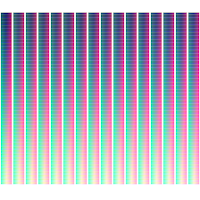VirtualDubffmpeg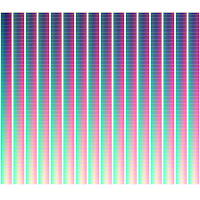Now to analyze, starting with some visual comparisons. Diffing and autoleveling (normalizing) exposes what pixels are different.

 Floating-point vs. VirtualDubFloating-point vs. ffmpeg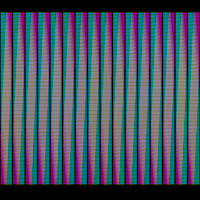Seems VirtualDub is far more accurate than ffmepg, but still doesn't match the floating-point version perfectly.

Now some numbers.
• VirtualDub has 1795792 pixels (11%) different from the floating-point conversion.
• ffmpeg has 10827725 pixels (65%) different from the floating-point conversion.
Differences broken down by color channel.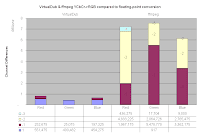I'm disappointed but not surprised that there are so many 1-off values in general. But ffmpeg's variance is as much as -3?? Wow, I hope I'm doing something wrong because that's pretty bad.

In the rare case someone has over an hour and 10GB to spare, along with various strange prerequisites, you can download the scripts used to generate these details.

*
`FFmpeg version SVN-r22107, Copyright (c) 2000-2010 the FFmpeg developers  built on Feb 28 2010 06:11:15 with gcc 4.4.2  configuration: --enable-memalign-hack --cross-prefix=i686-mingw32- --cc=ccache-i686-mingw32-gcc --arch=i686 --target-os=mingw32 --enable-runtime-cpudetect --enable-avisynth --enable-gpl --enable-version3 --enable-bzlib --enable-libgsm --enable-libfaad --enable-pthreads --enable-libvorbis --enable-libtheora --enable-libspeex --enable-libmp3lame --enable-libopenjpeg --enable-libxvid --enable-libschroedinger --enable-libx264 --enable-libopencore_amrwb --enable-libopencore_amrnb  libavutil     50. 9. 0 / 50. 9. 0  libavcodec    52.55. 0 / 52.55. 0  libavformat   52.54. 0 / 52.54. 0  libavdevice   52. 2. 0 / 52. 2. 0  libswscale     0.10. 0 /  0.10. 0`

## Saturday, March 13, 2010

### IDCT Demystified (a little)

The inverse discrete cosine transform is a very mysterious and intimidating equation.(apologies if I messed up the notation)

For the longest time I let the IDCT remain a black box. I found a handful of Java IDCT implementations, plugged them in, and cross my fingers.

I know what the 2D DCT does: it pushes all the image data to the top left corner of the block, while the IDCT undoes that magic. I'm not sure how it does this, but just knowing what it does is enough for me.

But recently I finally discovered that the IDCT is simply a couple of matrix multiplications.

idct_matrixT . coefficients . idct_matrix

The IDCT equation doesn't really suggest that to the casual mathematician. Of course if you take a class or pay for a book on the subject, maybe this is old news to you.

For those uninformed like me, let's take a closer look at this IDCT matrix.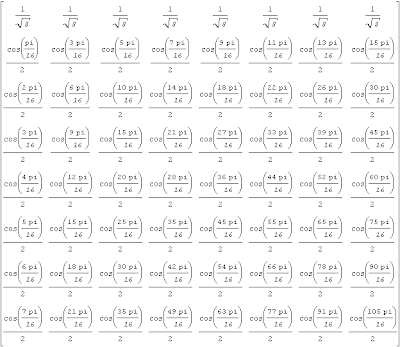Theoretically we could throw a bunch of trigonometry identities at this matrix to simplify it, but it turns out to be so much easier to just calculate it and see which decimal values are the same. In the end, there turns out to only be 7 unique values (listed here in varying forms).

`1/sqrt(8)       =  cos(  PI/ 4)/2cos(1*PI/16)/2  =  cos(  PI/16)/2  =  sqrt(2+sqrt(2+sqrt(2)))/4cos(2*PI/16)/2  =  cos(  PI/ 8)/2  =  sqrt(2+sqrt(2))/4cos(3*PI/16)/2  =  cos(3*PI/16)/2  =  sqrt(2+sqrt(2-sqrt(2)))/4cos(5*PI/16)/2  =  cos(5*PI/16)/2  =  sqrt(2-sqrt(2-sqrt(2)))/4cos(6*PI/16)/2  =  cos(3*PI/ 8)/2  =  sqrt(2-sqrt(2))/4cos(7*PI/16)/2  =  cos(7*PI/16)/2  =  sqrt(2-sqrt(2+sqrt(2)))/4`
Now the IDCT matrix can be simplified to this:Taking things a step further, let's multiply the two IDCT matrix multiplications out (Maxima is awesome). After a lot of trigonometric simplification, it turns into a massive matrix. This tiny portion below resembles what the entire matrix looks like.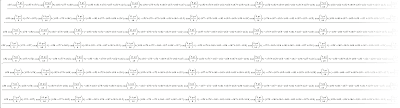You can download the full 30,000 pixel wide image if you dare.

All those additions/subtractions help to explain why fast IDCT implementations consist of so many sums and only occasional multiplications.

The bare math still makes it difficult to identify patterns, so I took things to the extreme and visualized it a bit.